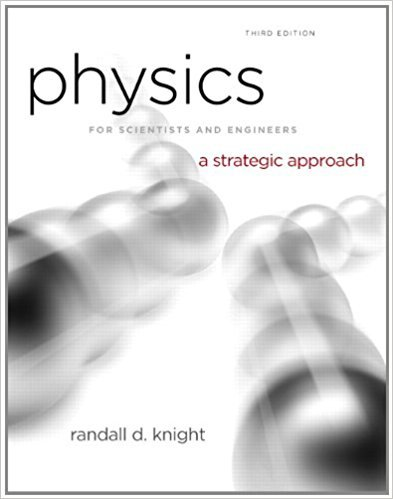×
Get Full Access to Physics For Scientists And Engineers: A Strategic Approach With Modern Physics - 3 Edition - Chapter 7 - Problem 57cp
Get Full Access to Physics For Scientists And Engineers: A Strategic Approach With Modern Physics - 3 Edition - Chapter 7 - Problem 57cp

×

# FIGURE shows three hanging masses connected by mass-lessISBN: 9780321740908 69

## Solution for problem 57CP Chapter 7

Physics for Scientists and Engineers: A Strategic Approach with Modern Physics | 3rd Edition

• Textbook Solutions
• 2901 Step-by-step solutions solved by professors and subject experts
• Get 24/7 help from StudySoup virtual teaching assistantsPhysics for Scientists and Engineers: A Strategic Approach with Modern Physics | 3rd Edition

4 5 1 329 Reviews
13
2
Problem 57CP

Problem 57CP

FIGURE shows three hanging masses connected by mass-less strings over two massless, frictionless pulleys.

a. Find the acceleration constraint for this system. It is a single equation relating a1y, a2y, and a3y.

Hint: yA isn’t constant.

b. Find an expression for the tension in string A.

Hint: You should be able to write four second-law equations. These, plus the acceleration constraint, are five equations in five unknowns.

c. Suppose: m1 = 2.5 kg, m2 = 1.5 kg, and m3 = 4.0 kg. Find the acceleration of each.

d. The 4.0 kg mass would appear to be in equilibrium. Explain why it accelerates.

FIGUREStep-by-Step Solution:

Solution

Step 1 of 6

In this problem, we have to find the acceleration constraint, expression for the tension, the acceleration of each for given masses and explain why acceleration.

Step 2 of 6

Step 3 of 6

##### ISBN: 9780321740908

Unlock Textbook Solution